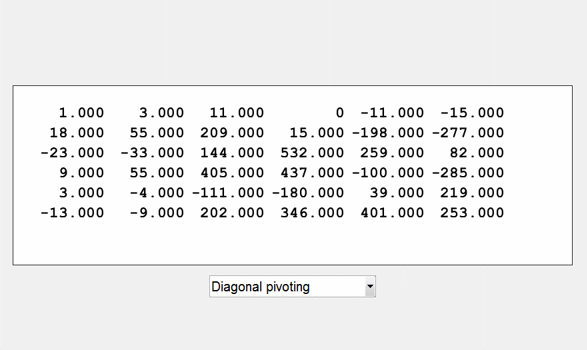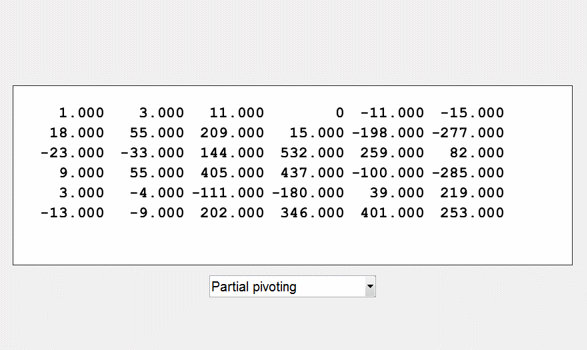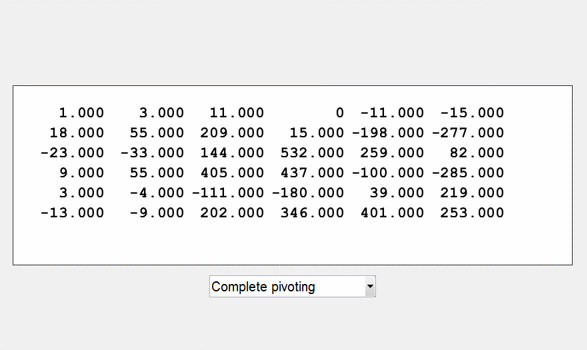# Golub Matrices, Deceptively Ill Conditioned

I got the idea for these test matrices from Gene Golub years ago, but the mathematical foundation comes from a paper by Divakar Viswanath and Nick Trefethen.

### Contents

#### Two programs from NCM

This post is about two MATLAB programs included in the collection from Numerical Computing with MATLAB. The program golub generates badly conditioned integer test matrices. The program lugui is an interactive graphical interface that allows you to experiment with pivot strategies in Gaussian elimination.

#### A 6-by-6 example

Set the random number generator to the state it has when MATLAB starts and generate a 6-by-6 example.
   rng('default')
A = golub(6)

A =
1     3    11     0   -11   -15
18    55   209    15  -198  -277
-23   -33   144   532   259    82
9    55   405   437  -100  -285
3    -4  -111  -180    39   219
-13    -9   202   346   401   253

It's not obvious that this matrix is badly conditioned, but it is.
   cond(A)

ans =
3.0115e+12

In case you needed another demonstration that the determinant does not indicate near singularity.
   format short
det(A)

ans =
1.0000

The elements of the inverse vary over nine orders of magnitude and show perverse scaling that is a direct consequence of the way this matrix was created.
   format short e
X = inv(A)

X =
2.8508e+09  -1.5513e+08   3.1110e+06   1.4854e+06  -1.6559e+05  -2.0380e+04
-1.3362e+09   7.2711e+07  -1.4582e+06  -6.9624e+05   7.7615e+04   9.5520e+03
1.0422e+08  -5.6713e+06   1.1373e+05   5.4306e+04  -6.0540e+03  -7.4500e+02
1.2586e+07  -6.8489e+05   1.3735e+04   6.5580e+03  -7.3100e+02  -9.0000e+01
-8.4225e+05   4.5833e+04  -9.1900e+02  -4.3900e+02   4.9000e+01   6.0000e+00
-1.3830e+05   7.5260e+03  -1.5100e+02  -7.2000e+01   8.0000e+00   1.0000e+00


#### Product of triangular factors

In a 1998 paper Divakar Viswanath and Nick Trefethen demonstrate that the 2-norm condition number of an n -by- n triangular matrix with normally distributed entries below the diagonal and ones on the diagonal grows exponentially almost surely at a rate c^n with c = 1.30568... I want my matrices to have integer entries so I use randomly distributed integers, but the exponential behavior must be nearly the same. Here is the code for golub.
   type golub

function A = golub(n)
%GOLUB  Badly conditioned integer test matrices.
%   GOLUB(n) is the product of two random integer n-by-n matrices,
%   one of them unit lower triangular and one unit upper triangular.
%   LU factorization without pivoting fails to reveal that such

%   Copyright 2014 The MathWorks, Inc.

s = 10;
L = tril(round(s*randn(n)),-1)+eye(n);
U = triu(round(s*randn(n)),1)+eye(n);
A = L*U;


#### Experiment with pivoting

Lugui lets you use the mouse to select the pivot elements for Gaussian elimination. Or, you can choose diagonal pivoting, partial pivoting or complete pivoting. Here are animated gifs of these three choices.#### Resurrecting ancestors

Diagonal pivoting actually defies the conventional wisdom and picks the smallest possible element at each stage as the pivot, namely 1.0. But this choice means that division by the pivot involves no roundoff. Moreover the choice leads to the reconstruction of the original triangular factors.
   [L,U] = lugui_noshow(A,'diagonal')
cond_L = cond(L)
cond_U = cond(U)

L =
1     0     0     0     0     0
18     1     0     0     0     0
-23    36     1     0     0     0
9    28    -2     1     0     0
3   -13    -1     7     1     0
-13    30    15    16    -8     1
U =
1     3    11     0   -11   -15
0     1    11    15     0    -7
0     0     1    -8     6   -11
0     0     0     1    11    24
0     0     0     0     1    -6
0     0     0     0     0     1
cond_L =
8.4806e+06
cond_U =
7.9889e+05


#### Diagonal of U

Partial pivoting gives only a weak indication that this matrix is badly conditioned.
   [L,U,p] = lugui_noshow(A,'partial');
condition_estimate_partial = max(abs(diag(U)))/min(abs(diag(U)))

condition_estimate_partial =
5.8208e+06

Complete pivoting does a much better job.
   [L,U,p,q] = lugui_noshow(A,'complete');
condition_estimate_complete = max(abs(diag(U)))/min(abs(diag(U)))

condition_estimate_complete =
1.5166e+12


#### Orthogonal factorization

The QR decomposition needs column pivoting to reveal the ill conditioning.
   [Q,R] = qr(A);
condition_estimate_without = max(abs(diag(R)))/min(abs(diag(R)))

[Q,R,E] = qr(A);
condition_estimate_with = max(abs(diag(R)))/min(abs(diag(R)))

condition_estimate_without =
6.6062e+06
condition_estimate_with =
2.2595e+12


#### References

Divakar Viswanath and Nick Trefethen, "Condition Numbers of Random Triangular Matrices," SIAM J. Matrix Anal. Appl. 19, 564-581, 1998, <http://epubs.siam.org/doi/pdf/10.1137/S0895479896312869>. Also available at https://people.maths.ox.ac.uk/trefethen/publication/PDF/1998_77.pdf Cleve Moler, Numerical Computing with MATLAB, 2004. https://www.mathworks.com/matlabcentral/fileexchange/37976-numerical-computing-with-matlab.

Published with MATLAB® R2015a
|